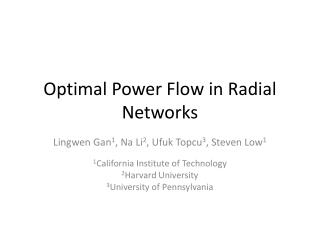DownloadDownload PresentationOptimal Power Flow in Radial Networks

# Optimal Power Flow in Radial Networks

Télécharger la présentation## Optimal Power Flow in Radial Networks

- - - - - - - - - - - - - - - - - - - - - - - - - - - E N D - - - - - - - - - - - - - - - - - - - - - - - - - - -
##### Presentation Transcript

1. Optimal Power Flow in Radial Networks Lingwen Gan1, Na Li2, Ufuk Topcu3, Steven Low1 1California Institute of Technology 2Harvard University 3University of Pennsylvania

2. Optimal power flow (OPF) What is OPF? Schedule power plants, minimize electricity cost. Is OPF important? Solved every 15 minutes, underlies power system operation.

3. Challenges in solving OPF Power flow governed by nonlinear (nonconvex) laws Three ways to deal with the challenge 1. Linearization / Approximation. [DC, …] 2. Local optima [Newton-Raphson, SQP, …] May guarantee global optimality 3. Convex relaxation [SDP, SOCP]

4. Convex relaxations of OPF Feasible set of OPF OPF is Quadratic Constrained Quadratic Programming Feasible set of SDP optimal solution standard relaxation Semi-Definite Programming (SDP) [Bai08, Javad10] Feasible set of SOCP radial network Second-Order Cone Programming (SOCP) [Farivar11, Javad12] Sufficient conditions derived for exact convex relaxation.

5. Outline Formulate OPF. Sufficient condition for exact SOCP. Modify OPF accordingly. Numerical case studies.

6. Terminologies in power network Power network represented by a graph. bus line Radial j i

7. The OPF problem min generation cost j i s S l v over power flow constraints s.t. Nonconvex! injection constraints voltage constraints

8. The SOCP relaxation OPF SOCP [Farivar11] feasible set of SOCP feasible set of OPF optimal solution Def: SOCP is exact  (every) optimal solution feasible for OPF.

9. When is relaxation exact? Counter examples exist. j i Sufficient conditions derived for radial networks. Type 1: Type 2: Type 3: s satisfy some (mild) constraints.

10. A sufficient condition Theorem 1: C1 + C2  SOCP exact. C1: C2: What is C1? Introduce for every line. root Impose .

11. A sufficient condition Theorem 1: C1 + C2  SOCP exact. C1: C2: is affine in s. SOCP if line loss is small.

12. Interpretation of C1 and C2 C1 + C2  SOCP exact. Hold for various networks with sufficient margin. Impose C2 in the first place.

13. A modified OPF Impose C2 in the first place. OPF-m Theorem: C1  SOCP exact for OPF-m.

14. Numerical studies C1  SOCP exact for OPF-m. How widely does C1 hold? How big is the modification? IEEE 13-, 34-, 37-, 123-bus networks; SCE147-, 56-bus networks. 1Southern California Edison, a utility company in southern California, USA.

15. C1 holds with sufficient margin Yes C1 holds? No margin > 1  C1 holds. distributed generation & shunt capacitor larger margin  C1 holds more “safely.” C1 margin margin > 1 Without distributed generation, margin is very big (>10). With big distributed generation, margin is still sufficient.

16. OPF-m is similar to OPF The value ε quantifies the gap between OPF-m and OPF.

17. Summary C1 Checked a priori. Holds for all test networks. + power power current C2 Motivate modified OPF problem. SOCP is exact